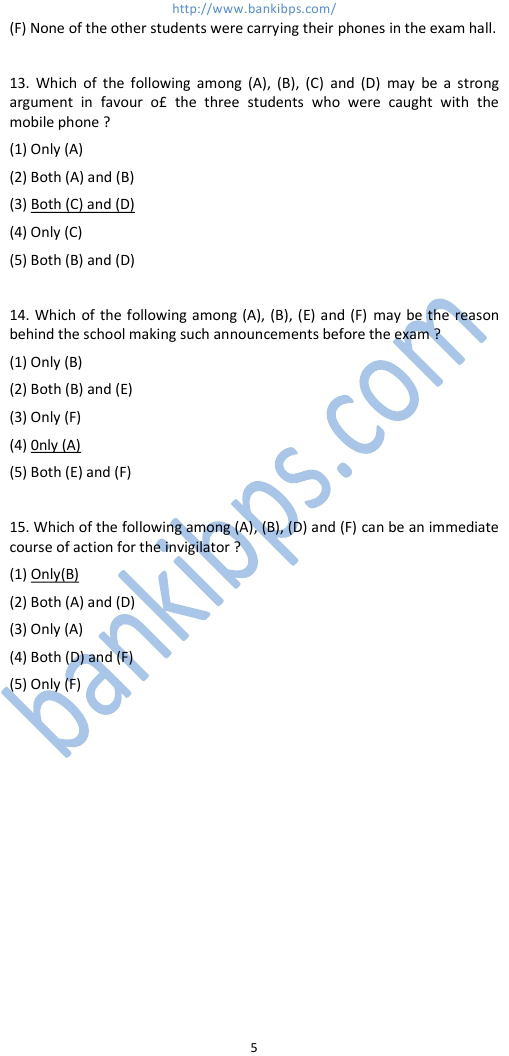# SBI PO 2013 Solution

Directions (6-8) : Each of the questions below consists of a question and two statements numbered I and II given below it. You have to decide whether the data given in the statements are sufficient to answer the questions. Read bath the statements and — Give answer (1) if the data in statement I alone are sufficient to answer the question, while the data in statement II alone are not sufficient to answer the question. Give answer (2) if the data in statement II alone are sufficient to answer the question, while the data in statement I alone are not sufficient to answer the question. Give answer (3) if the data either in statement I alone or statement II alone are sufficient to answer the question. Give answer (4) if the data neither in statement l nor II together are sufficient to answer the questions. Give answer (5) if the data in both statements I and II together are necessary to answer the questions. sbi po 2013 solution 6. Seventeen people are standing in a straight line facing south. What is Bhavna’s position from the left end of the line ? I. Sandeep is standing second to the left of Sheetal. Only five people stand between Sheetal and the one who is standing at the extreme right end of the line. Four people stand between Sandeep and Bhavna. II. Anita is standing fourth to the left of Sheetal. Less than three people are standing between Bhavna and Anita. Answer :1 7. Five letters -A, E, G, N and R are arranged left to right , according to certain conditions. Which letter is placed third. G is placed second to the right of A. E is to the immediate right of G. There are only two letters between R and G. II. N is exactly between A and G. Neither A nor G is. I at the extreme end of the arrangement. Answer : 5 8. Six people — S, T, U, V, W and X are sitting around a circular table facing the centre. What is T’s position with respect to X ? I. Only two people sit between U and W. X is second to the left of W. V and T are immediate neighbours of each other.
Reasoning